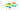## Concise.org

So we have our hypothesis function and we have a way of measuring (the cost function) how well it fits into the data. Now we need to estimate the parameters ($\theta_0$ and $\theta_1$) in hypothesis function. That's where gradient descent comes in.

We put $\theta_0$ on the x-axis and $\theta_1$ on the y-axis, with the cost function on the vertical z-axis. We need to find the specific theta parameters for which our cost function is at the very bottom of the pits in our graph, i.e. when its value is the minimum.

Gradient descent is a more general algorithm that can be used to minimize an arbitrary function $J$.

We make steps down the cost function in the direction with the steepest descent, and the size of each step is determined by the parameter $\alpha$, which is called the learning rate.

On a side note, we should adjust our parameter $\alpha$ to ensure that the gradient descent algorithm converges in a reasonable time. If $\alpha$ is too small, gradient decent can be slow to converge. On the other hand, if $\alpha$ is too large value, gradient descent can overshoot the minimum and it may fail to converge, or even diverge. Sometimes, if $\alpha$ is too large, slow convergence is also possible.

Even with a fixed learning rate $\alpha$, as we approach a local minimum, gradient descent will automatically take smaller steps. At the minima, the derivative will always be 0 and thus the $\theta$ values will no longer change.

repeat until convergence: {

$\,\,\,\,\,\, \theta_j := \theta_j - \alpha \frac{\partial}{\partial \theta_j} J(\theta_0, \theta_1) \,\,\,\,\,\,$ (simultaneously update for $j=0$ and $j=1$)

}

Note that $\theta_0$ and $\theta_1$ should be updated simultaneously.$\frac{\partial}{\partial \theta_j} J(\theta_0, \theta_1)$ is the slope of tangent also known as derivative in j dimension.

In higher dimensions, the analog of derivative is gradient. The gradient measures the vector direction of steepest descent of the function.

Depending on where one starts on the graph, one could end up at different points.